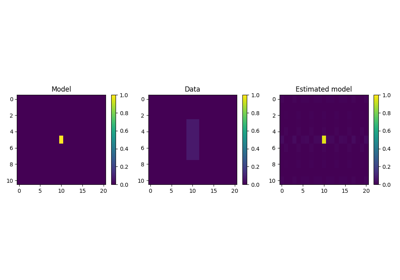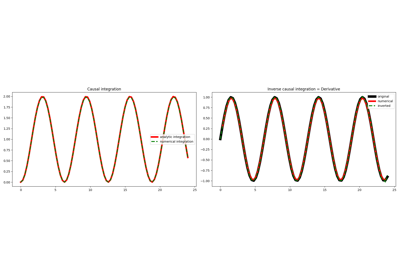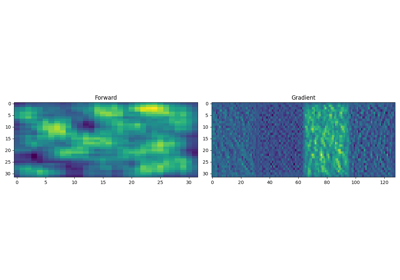# pylops.Smoothing2D¶

pylops.Smoothing2D(nsmooth, dims, axes=(-2, -1), dtype='float64')[source]

2D Smoothing.

Apply smoothing to model (and data) along two axes of a multi-dimensional array.

Parameters
nsmooth

Lenght of smoothing operator in 1st and 2nd dimensions (must be odd)

dimstuple

Number of samples for each dimension

axesint, optional

New in version 2.0.0.

Axes along which model (and data) are smoothed.

dtypestr, optional

Type of elements in input array.

Notes

The 2D Smoothing operator is a special type of convolutional operator that convolves the input model (or data) with a constant 2d filter of size $$n_{\text{smooth}, 1} \times n_{\text{smooth}, 2}$$:

Its application to a two dimensional input signal is:

$y[i,j] = 1/(n_{\text{smooth}, 1}\cdot n_{\text{smooth}, 2}) \sum_{l=-(n_{\text{smooth},1}-1)/2}^{(n_{\text{smooth},1}-1)/2} \sum_{m=-(n_{\text{smooth},2}-1)/2}^{(n_{\text{smooth},2}-1)/2} x[l,m]$

Note that since the filter is symmetrical, the Smoothing2D operator is self-adjoint.

Attributes
shapetuple

Operator shape

explicitbool

Operator contains a matrix that can be solved explicitly (True) or not (False)

## Examples using pylops.Smoothing2D¶2D Smoothing

2D SmoothingCausal Integration

Causal Integration19. Automatic Differentiation

19. Automatic Differentiation